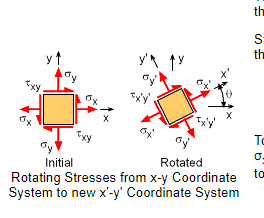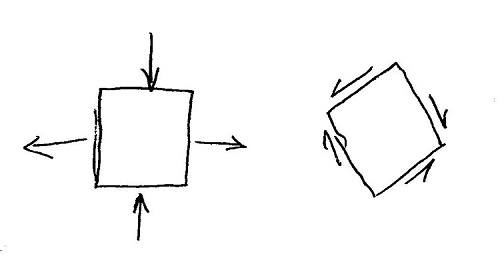# Principle Stress Planes

Kajan thana
TL;DR Summary
I am struggling to understand the concept of Principle Stress plane.
All the materials that I have been reading, it says that when the element is rotated to a θ, this will be called the principle stress plane with no shear force acting on it. but visually when you rotate the element, there will be a shear force acting parallel to the plane. I am very confused, can someone help me, please? Do we have to assume that at some θ, there will be no shear force acting?#### Attachments

Mentor
No assumption needed, but start by going back to the simplest cases. Start with the case below, and assume that all normal forces are equal. Study the transformation to the 45 degree rotated case, where the normal forces are zero, and there are four equal shear stresses. Then start with the case of four equal shear stresses without normal stresses, and rotate another 45 degrees. Repeat until it all makes sense.

Then study the equations until you figure out from the equations how far to rotate to get from pure normal to pure shear stress, and vice versa. You know from inspection that the rotation will be 45 degrees, but you need to understand how to find that angle from the equations.The second simplest case is a normal stress in one direction, and zero stress in the perpendicular direction. Study rotations of that case until it all makes sense. Hint: The angle for pure shear will not be 45 degrees. After all of that is clear in your mind, the more complex cases should make sense because the most complex cases are the superposition of the simple cases.

This is a fundamental concept, and it is well worth the time it takes to fully understand it.

•Kajan thana
Mentor
There are three orientations of differential surfaces at each location within the material for which the stress vector on the surface (aka traction vector) acts purely normal to the surface.

•Kajan thana
Kajan thana
Thank you so much, It makes much more sense. Appreciate your help.

•jrmichler